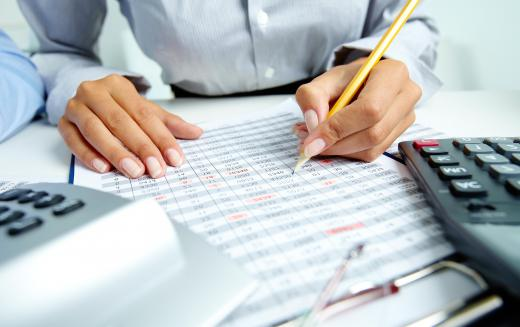Finance
Fact Checked

# What is Depreciation Expense?

Lea Miller
Lea Miller

Depreciation expense is the percentage of the total value of a fixed asset that is determined to have been depleted during a specific accounting period. The purpose of depreciation expense is to gradually devalue items as they age and sustain wear and tear through regular use. Types of depreciation methods include straight-line, sum-of-the-years, declining balance, and units of production.

When a business makes a significant purchase such as a vehicle or machine, the item is recorded as a fixed asset and an evaluation is made to determine the expected term of use for the asset. The item is depreciated over the years of its useful life to reflect the percentage of its value in each accounting period. Consumable items are considered expenses at the time of purchase. A piece of machinery with an expected life of five years, for example, will be depreciated. The oil or gaskets used to maintain the machine are recorded as expenses immediately because they are used for only a short time and then replaced.Depreciation expense is the percentage of the total value of a fixed asset that is determined to have been depleted during a specific accounting period.

Depreciation expense is considered to be a non-cash item because it does not require a cash outlay in a given accounting period. The cash disbursement occurs at the time the asset was purchased. In order to display a consistent profit and loss statement for monthly accounting periods, annual depreciation expense is divided by twelve and posted as a monthly expense.

The simplest method of depreciation is straight-line. The value of the asset less any expected salvage value is divided by the number of years of its expected useful life. For an asset with a five year expected life, the depreciation expense will be 20% of the depreciable value each year.

The calculation for the method called sum-of-the-years-digits is based on fractional amounts. This depreciation method shifts more expense to earlier years than the straight-line method. For an asset with a useful life of five years, the depreciation is divided into fractional quantities based on the sum of the numbers for each year (1 + 2 + 3 + 4 + 5 = 15). The depreciation recorded is 5/15 of the value in the first year, 4/15 in the second year, and so on.

The declining balance method shifts an even larger amount of depreciation into earlier periods. It is typically calculated with double the straight-line rate and is therefore called the double declining balance method. The formula to calculate double declining balance depreciation for a given year is to subtract the accumulated depreciation from the original value and then divide by the useful life in years and multiply by two. Salvage value is deducted in the final year.

Another depreciation method is based on units of production. This depreciation formula subtracts the salvage value from the original value and then divides by the total expected production units and multiplies by the actual number of production units for the period. This method ties the depreciation expense recorded to the actual production levels for the accounting period.

## You might also Like